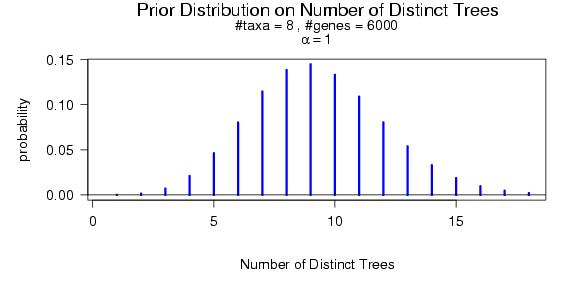## Choice of the a priori discordance parameter α

α=0 corresponds to the a priori assumption that there is no discordance among gene tree topologies, i.e. that all loci must have the same topology.
α=infinity corresponds to the a priori assumption that gene trees are completely independent, as in a consensus approach.

### Prior probability that two genes share the same topoloty

The prior probability that two randomly sampled genes share the same tree is approximately p=1/(α+1) .
The exact value of this probability depends on the total number T of a priori possible topologies:
p=(1+α/T)/(α+1) .

### Prior distribution on the number of distinct trees

You can visualize this distribution using R as follows.